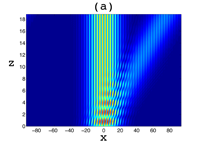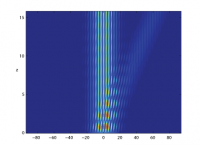Analytic Solution for PT-Symmetric Volume Gratings

Mykola Kulishov, H. F. Jones, Bernard Kress

We study the diffraction produced by a PT-symmetric volume Bragg grating that combines modulation of refractive index and gain/loss of the same periodicity with a quarter-period shift between them. Such a complex grating has a directional coupling between the different diffraction orders, which allows us to find an analytic solution for the first three orders of the full Maxwell equations without resorting to the paraxial approximation. This is important, because only with the full equations can the boundary conditions, allowing for reflections, be properly implemented. Using our solution we analyze the properties of such a grating in a wide variety of configurations.

http://arxiv.org/abs/1412.0506
Optics (physics.optics)

Singular Mapping for a PT-Symmetric Sinusoidal Optical Lattice at the Symmetry-Breaking Threshold

H. F. Jones

A popular PT-symmetric optical potential (variation of the refractive index) that supports a variety of interesting and unusual phenomena is the imaginary exponential, the limiting case of the potential $$V_0[\cos(2\pi x/a)+i\lambda\sin(2\pi x/a)]$$ as $$\lambda\to1$$, the symmetry-breaking point. For $$\lambda<1$$, when the spectrum is entirely real, there is a well-known mapping by a similarity transformation to an equivalent Hermitian potential. However, as $$\lambda\to1$$, the spectrum, while remaining real, contains Jordan blocks in which eigenvalues and the corresponding eigenfunctions coincide. In this limit the similarity transformation becomes singular. Nonetheless, we show that the mapping from the original potential to its Hermitian counterpart can still be implemented; however, the inverse mapping breaks down. We also illuminate the role of Jordan associated functions in the original problem, showing that they map onto eigenfunctions in the associated Hermitian problem.

http://arxiv.org/abs/1411.6451
Optics (physics.optics); Mathematical Physics (math-ph); Quantum Physics (quant-ph)

Unique optical characteristics of a Fabry-Perot resonator with embedded PT-symmetrical grating

Mykola Kulishov, Bernand Kress, H. F. Jones

We explore the optical properties of a Fabry-Perot resonator with an embedded Parity-Time (PT) symmetrical grating. This PT-symmetrical grating is non diffractive (transparent) when illuminated from one side and diffracting (Bragg reflection) when illuminated from the other side, thus providing a unidirectional reflective functionality. The incorporated PT-symmetrical grating forms a resonator with two embedded cavities. We analyze the transmission and reflection properties of these new structures through a transfer matrix approach. Depending on the resonator geometry these cavities can interact with different degrees of coherency: fully constructive interaction, partially constructive interaction, partially destructive interaction, and finally their interaction can be completely destructive. A number of very unusual (exotic) nonsymmetrical absorption and amplification behaviors are observed. The proposed structure also exhibits unusual lasing performance. Due to the PT-symmetrical grating, there is no chance of mode hopping; it can lase with only a single longitudinal mode for any distance between the distributed reflectors.

http://arxiv.org/abs/1405.6024
Optics (physics.optics)

The Floquet Method for PT-symmetric Periodic Potentials

H. F. Jones

By the general theory of PT-symmetric quantum systems, their energy levels are either real or occur in complex-conjugate pairs, which implies that the secular equation must be real. However, for periodic potentials it is by no means clear that the secular equation arising in the Floquet method is indeed real, since it involves two linearly independent solutions of the Schrodinger equation. In this brief note we elucidate how that reality can be established.

http://arxiv.org/abs/1211.1560
Mathematical Physics (math-ph); Quantum Physics (quant-ph)

Analytic Results for a PT-symmetric Optical Structure

H. F. Jones

Propagation of light through media with a complex refractive index in which gain and loss are engineered to be PT symmetric has many remarkable features. In particular the usual unitarity relations are not satisfied, so that the reflection coefficients can be greater than one, and in general are not the same for left or right incidence. Within the class of optical potentials of the form $$v(x)=v_1\cos(2\beta x)+iv_2\sin(2\beta x)$$ the case $$v_2=v_1$$ is of particular interest, as it lies on the boundary of PT-symmetry breaking. It has been shown in a recent paper by Lin et al. that in this case one has the property of “unidirectional invisibility”, while for propagation in the other direction there is a greatly enhanced reflection coefficient proportional to $$L^2$$, where $$L$$ is the length of the medium in the direction of propagation.

For this potential we show how analytic expressions can be obtained for the various transmission and reflection coefficients, which are expressed in a very succinct form in terms of modified Bessel functions. While our numerical results agree very well with those of Lin et al. we find that the invisibility is not quite exact, in amplitude or phase. As a test of our formulas we show that they identically satisfy a modified version of unitarity appropriate for PT-symmetric potentials. We also examine how the enhanced transmission comes about for a wave-packet, as opposed to a plane wave, finding that the enhancement now arises through an increase, of $$O(L)$$, in the pulse length, rather than the amplitude.

http://arxiv.org/abs/1111.2041
Optics (physics.optics); Quantum Physics (quant-ph)

PT-Symmetric Sinusoidal Optical Lattices at the Symmetry-Breaking Threshold

Eva-Maria Graefe, H. F. JonesThe PT-symmetric potential $$V_0[\cos(2\pi x/a)+i\lambda\sin(2\pi x/a)]$$ has a completely real spectrum for $$\lambda\le 1$$, and begins to develop complex eigenvalues for $$\lambda>1$$. At the symmetry-breaking threshold $$\lambda=1$$ some of the eigenvectors become degenerate, giving rise to a Jordan-block structure for each degenerate eigenvector. In general this is expected to result in a secular growth in the amplitude of the wave. However, it has been shown in a recent paper by Longhi, by numerical simulation and by the use of perturbation theory, that for a broad initial wave packet this growth is suppressed, and instead a saturation leading to a constant maximum amplitude is observed. We revisit this problem by explicitly constructing the Bloch wave-functions and the associated Jordan functions and using the method of stationary states to find the dependence on the longitudinal distance $$z$$ for a variety of different initial wave packets. This allows us to show in detail how the saturation of the linear growth arises from the close connection between the contributions of the Jordan functions and those of the neighbouring Bloch waves.

http://arxiv.org/abs/1104.2838
Optics (physics.optics); Quantum Physics (quant-ph)

Use of Equivalent Hermitian Hamiltonian for PT-Symmetric Sinusoidal Optical Lattices

H. F. JonesWe show how the band structure and beam dynamics of non-Hermitian $PT$-symmetric sinusoidal optical lattices can be approached from the point of view of the equivalent Hermitian problem, obtained by an analytic continuation in the transverse spatial variable $x$. In this latter problem the eigenvalue equation reduces to the Mathieu equation, whose eigenfunctions and properties have been well studied. That being the case, the beam propagation, which parallels the time-development of the wave-function in quantum mechanics, can be calculated using the equivalent of the method of stationary states. We also discuss a model potential that interpolates between a sinusoidal and periodic square well potential, showing that some of the striking properties of the sinusoidal potential, in particular birefringence, become much less prominent as one goes away from the sinusoidal case.

http://arxiv.org/abs/1009.5784
Optics (physics.optics); Quantum Physics (quant-ph)